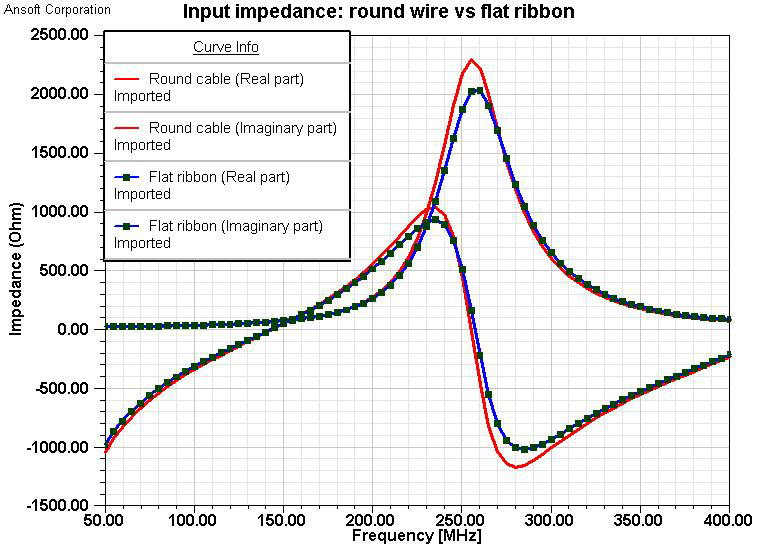Evaluation of Electromagnetic Modeling Tools, January 2009

Modeling the Dipole as a Round Wire or Flat Ribbon

Modeling a Center-driven Dipole with HFSS

The round wire can be approximated by a narrow, flat ribbon. The flat ribbon has the same length, zero thickness, and an equivalent width. The relationship between the equivalent width w of the flat ribbon and the radius a of a round wire is derived by assuming that the surface current density is uniformly distributed on the effective surface of the two dipole arms .

w = 4 ×a

The input impedance of a 0.5-m long dipole was calculated using these two models. The results are plotted in Figure 1.Fig. 1. Input impedances of round wire and flat ribbon models

The input impedances of the two models, 0.5-mm round wire and 2-mm wide flat ribbon, are almost the same over the frequency range evaluated. Also, we compared the results in the following table.

 Round wire (a = 1 mm) Flat ribbon (w = 2 mm) Impedance at 150 MHz (Ohm) 71.9+j46.7 71.8+j48.8 The first resonance frequency (MHz) 144 143 Simulation time 3 mins 47 secs 2 mins 46 secs Number of tetrahedra 14073 7479

Depending on the radius of the wire, the length of the dipole for the first resonance is about L = 0.47 to 0.48 wavelengths . For a 1-m dipole, the first resonance frequency should be between 141 MHz and 144 MHz. Using the flat ribbon model, both the input impedance and the resonance frequency are close to the theoretical values.

Reference:

 C. Balanis, Antenna Theory: Analysis and Design, pp. 403-412, pp. 449-453, John Wiley & Sons, Inc., New York, 2001.